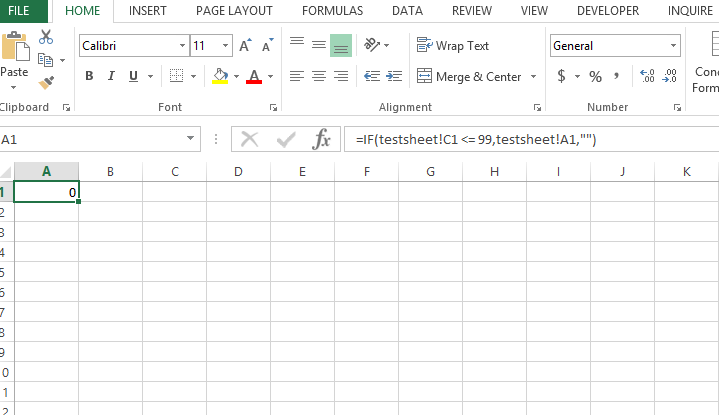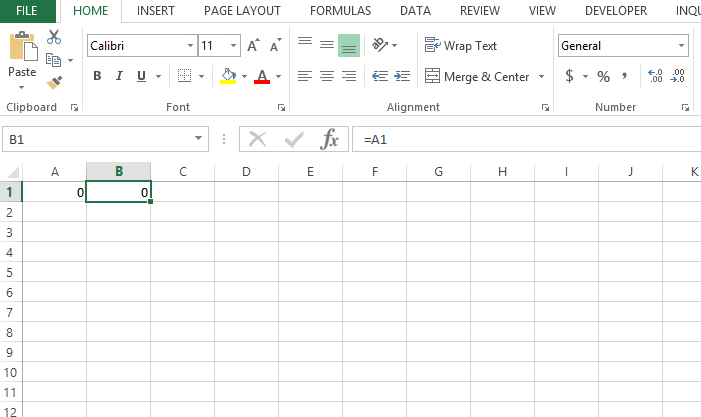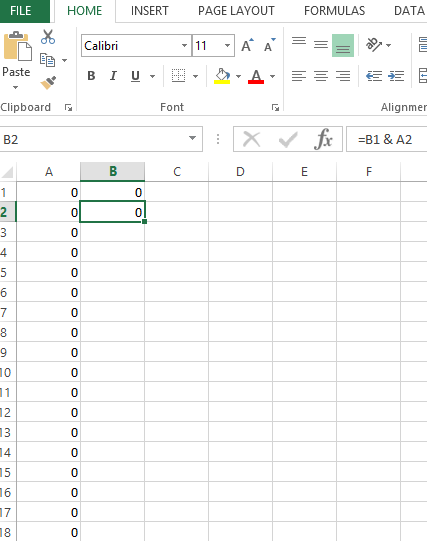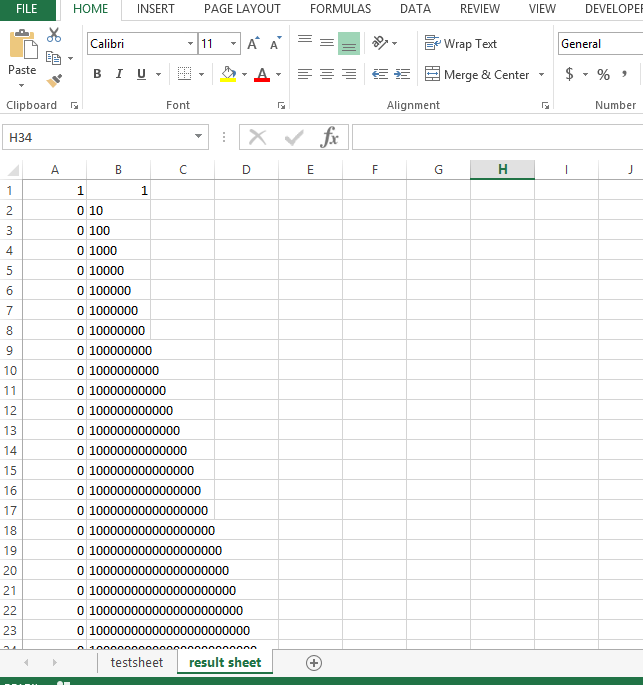#### How to loop without VBA or macros?

Looping is a common task in Excel, and it can be done using VBA or macros. However, there are also ways to loop in Excel without using VBA or macros.

In this article, we will show you how to loop in Excel using the `IF` function and the `INDEX` function.

It is very common to create the required loops in Excel vba like if loop, do while loop, do until loop, for loop, while wend loop for different purposes.

But here we will create a similar effect in Excel using formulas.

## Excel loop formula

In any Excel file name the sheet as “testsheet” and “result sheet” and in cell A1 of “result sheet” white formula:

=IF(‘testsheet’!C1 <= 99,’testsheet’!A1,””)

Copy this until row 40.Now in cell B1 write formula: =A1Now in cell B2 write: =B1 & A2## Looping in Excel without Vba code

Copy this till B40. We will get the following results:It worked the same as for loop in but without Vba code involved.

## How to Loop Using the `IF` Function and the `INDEX` Function

To loop using the `IF` function and the `INDEX` function, you will need to:

1. Create a list of the values you want to loop through.
2. Give the list a descriptive name, such as “Values.”
3. In the first cell where you want to display the first value in your list, enter the formula `=INDEX(Values,1)`.
4. In the next cell, enter the formula `=IF(INDEX(Values,ROW()-1)<>INDEX(Values,ROWS(Values)),INDEX(Values,ROW()),"")`.
5. Copy the formula down the column.

The `IF` function in this formula checks if the current value is different from the previous value. If it is, the current value is displayed in the cell. If it is not, the cell is left blank.

The `INDEX` function in this formula retrieves the current value from the list.

### Example

Let’s say we have a list of numbers in cells A1 to A10. We want to loop through this list and display the numbers one by one in cells B1 to B10.

We would first create a named range for the list of numbers. We could do this by selecting the cells A1 to A10 and then pressing `Ctrl`+`F3`. In the “Name” box, we would type “Values” and then click on “OK”.

Next, we would enter the formula `=INDEX(Values,1)` in cell B1.

Finally, we would copy the formula down column B.

The result would be that the numbers in cells A1 to A10 would be displayed in cells B1 to B10, one by one.

## Conclusion

Looping is a powerful tool that can be used to automate tasks in Excel. In this article, we have shown you how to loop in Excel using the `IF` function and the `INDEX` function. This is a simple and effective way to loop in Excel without using VBA or macros.

Here are some additional tips for looping in Excel:

• Use the `FOR` loop to loop a specific number of times.
• Use the `WHILE` loop to loop until a certain condition is met.
• Use the `DO...LOOP` loop to loop until a certain condition is met or a certain number of times is reached.
• Use the `EXIT` statement to exit a loop early.